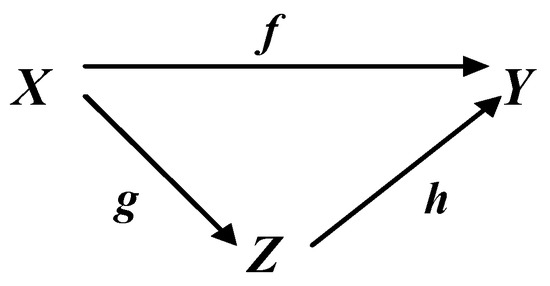Previous Article in Journal
A Symmetry-Breaking Node Equivalence for Pruning the Search Space in Backtracking Algorithms
Previous Article in Special Issue
The Influence of a Network’s Spatial Symmetry, Topological Dimension, and Density on Its Percolation Threshold
Open AccessArticle

# A General Principle of Isomorphism: Determining Inverses

byVladimir S. Kulabukhov
Uspensky Avionica Moscow Research and Production Complex JSC; 7, Obraztsov St. 127055 Moscow, Russia
Symmetry 2019, 11(10), 1301; https://doi.org/10.3390/sym11101301
Received: 18 July 2019 / Revised: 20 September 2019 / Accepted: 9 October 2019 / Published: 15 October 2019
The problem of determining inverses for maps in commutative diagrams arising in various problems of a new paradigm in algebraic system theory based on a single principle—the general principle of isomorphism is considered. Based on the previously formulated and proven theorem of realization, the rules for determining the inverses for typical cases of specifying commutative diagrams are derived. Simple examples of calculating the matrix maps inverses, which illustrate both the derived rules and the principle of relativity in algebra based on the theorem of realization, are given. The examples also illustrate the emergence of new properties (emergence) in maps in commutative diagrams modeling (realizing) the corresponding systems. View Full-Text
Show FiguresFigure 1

MDPI and ACS Style

Kulabukhov, V.S. A General Principle of Isomorphism: Determining Inverses. Symmetry 2019, 11, 1301.